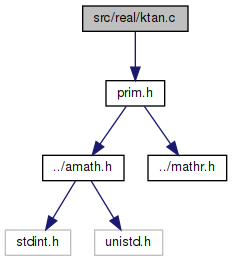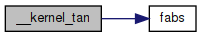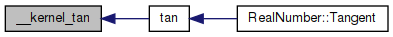amath  1.8.5 Simple command line calculator
ktan.c File Reference

Kernel tan function. More...

`#include "prim.h"`
Include dependency graph for ktan.c:Go to the source code of this file.

## Macros

#define one   xxx

#define pio4   xxx

#define pio4lo   xxx

#define T   xxx

## Functions

double __kernel_tan (double x, double y, int iy)
Kernel tan function. More...

## Variables

static const double xxx []

## Detailed Description

Kernel tan function.

Definition in file ktan.c.

## ◆ one

 #define one   xxx

Definition at line 67 of file ktan.c.

## ◆ pio4

 #define pio4   xxx

Definition at line 68 of file ktan.c.

## ◆ pio4lo

 #define pio4lo   xxx

Definition at line 69 of file ktan.c.

## ◆ T

 #define T   xxx

Definition at line 70 of file ktan.c.

## ◆ __kernel_tan()

 double __kernel_tan ( double x, double y, int iy )

Kernel tan function.

```Kernel tan function on [-pi/4, pi/4], pi/4 ~ 0.7854
Input x is assumed to be bounded by ~pi/4 in magnitude.
Input y is the tail of x.
Input k indicates whether tan (if k = 1) or -1/tan (if k = -1) is returned.```
```Algorithm
1. Since tan(-x) = -tan(x), we need only to consider positive x.
2. if x < 2^-28 (hx<0x3e300000 0), return x with inexact if x!=0.
3. tan(x) is approximated by a odd polynomial of degree 27 on
[0,0.67434]
3             27
tan(x) ~ x + T1*x + ... + T13*x
where
|tan(x)         2     4            26   |     -59.2
|----- - (1+T1*x +T2*x +.... +T13*x    )| <= 2
|  x                    |
```
```    Note: tan(x+y) = tan(x) + tan'(x)*y
~ tan(x) + (1+x*x)*y
Therefore, for better accuracy in computing tan(x+y), let
3      2      2       2       2
r = x *(T2+x *(T3+x *(...+x *(T12+x *T13))))
then
3    2
tan(x+y) = x + (T1*x + (x *(r+y)+y))```
```     4. For x in [0.67434,pi/4],  let y = pi/4 - x, then
tan(x) = tan(pi/4-y) = (1-tan(y))/(1+tan(y))
= 1 - 2*(tan(y) - (tan(y)^2)/(1+tan(y)))
```

Definition at line 108 of file ktan.c.

References fabs().

Referenced by tan().

109 {
110  double z, r, v, w, s;
111  double a, t;
112  int32_t ix, hx;
113  uint32_t low;
114
115  // high word of x
116  GET_HIGH_WORD(hx, x);
117
118  // high word of |x|
119  ix = hx & 0x7FFFFFFF;
120
121  // x < 2**-28
122  if (ix < 0x3E300000)
123  {
124  // generate inexact
125  if ((int32_t)x == 0)
126  {
127  GET_LOW_WORD(low, x);
128
129  if (((ix | low) | (iy + 1)) == 0)
130  {
131  return one / fabs(x);
132  }
133
134  if (iy == 1)
135  {
136  return x;
137  }
138
139  // compute -1 / (x+y) carefully
140  z = w = x + y;
141  SET_LOW_WORD(z, 0);
142  v = y - (z - x);
143  t = a = -one / w;
144  SET_LOW_WORD(t, 0);
145  s = one + t * z;
146  return t + a * (s + t * v);
147  }
148  }
149
150  // |x| >= 0.6744
151  if (ix >= 0x3FE59428)
152  {
153  if (hx < 0)
154  {
155  x = -x;
156  y = -y;
157  }
158  z = pio4 - x;
159  w = pio4lo - y;
160  x = z + w;
161  y = 0.0;
162  }
163
164  z = x * x;
165  w = z * z;
166
167  /*
168  * Break x^5*(T+x^2*T+...) into
169  * x^5(T+x^4*T+...+x^20*T) +
170  * x^5(x^2*(T+x^4*T+...+x^22*[T12]))
171  */
172  r = T + w * (T + w * (T + w * (T + w * (T + w * T))));
173  v = z * (T + w * (T + w * (T + w * (T + w * (T + w * T)))));
174  s = z * x;
175  r = y + z * (s * (r + v) + y);
176  r += T * s;
177  w = x + r;
178
179  if (ix >= 0x3FE59428)
180  {
181  v = (double)iy;
182  return (double)(1 - ((hx >> 30) & 2)) *
183  (v - 2.0 * (x - (w * w / (w + v) - r)));
184  }
185
186  if (iy == 1)
187  {
188  return w;
189  }
190
191  // compute -1.0 / (x+r) accurately
192  z = w;
193  SET_LOW_WORD(z, 0);
194  v = r - (z - x); // z+v = r+x
195  t = a = -1.0 / w; // a = -1.0/w
196  SET_LOW_WORD(t, 0);
197  s = 1.0 + t * z;
198  return t + a * (s + t * v);
199 }
#define pio4
Definition: ktan.c:68
#define GET_HIGH_WORD(i, d)
Get the more significant 32 bit int from a double.
Definition: prim.h:167
#define pio4lo
Definition: ktan.c:69
#define GET_LOW_WORD(i, d)
Get the less significant 32 bit int from a double.
Definition: prim.h:177
#define T
Definition: ktan.c:70
#define SET_LOW_WORD(d, v)
Set the less significant 32 bits of a double from an int.
Definition: prim.h:209
#define one
Definition: ktan.c:67
double fabs(double x)
Returns the absolute value of x.
Definition: fabs.c:51
Here is the call graph for this function:Here is the caller graph for this function:## ◆ xxx

 const double xxx[]
static
Initial value:
= {
3.33333333333334091986e-01,
1.33333333333201242699e-01,
5.39682539762260521377e-02,
2.18694882948595424599e-02,
8.86323982359930005737e-03,
3.59207910759131235356e-03,
1.45620945432529025516e-03,
5.88041240820264096874e-04,
2.46463134818469906812e-04,
7.81794442939557092300e-05,
7.14072491382608190305e-05,
-1.85586374855275456654e-05,
2.59073051863633712884e-05,
1.00000000000000000000e+00,
7.85398163397448278999e-01,
3.06161699786838301793e-17
}

Definition at line 48 of file ktan.c.